# Geometry Expressions

## Center of Curvature

We generate code for the center of curvature of a curve.

First create a parametric curve with X=f(T), Y=g(T).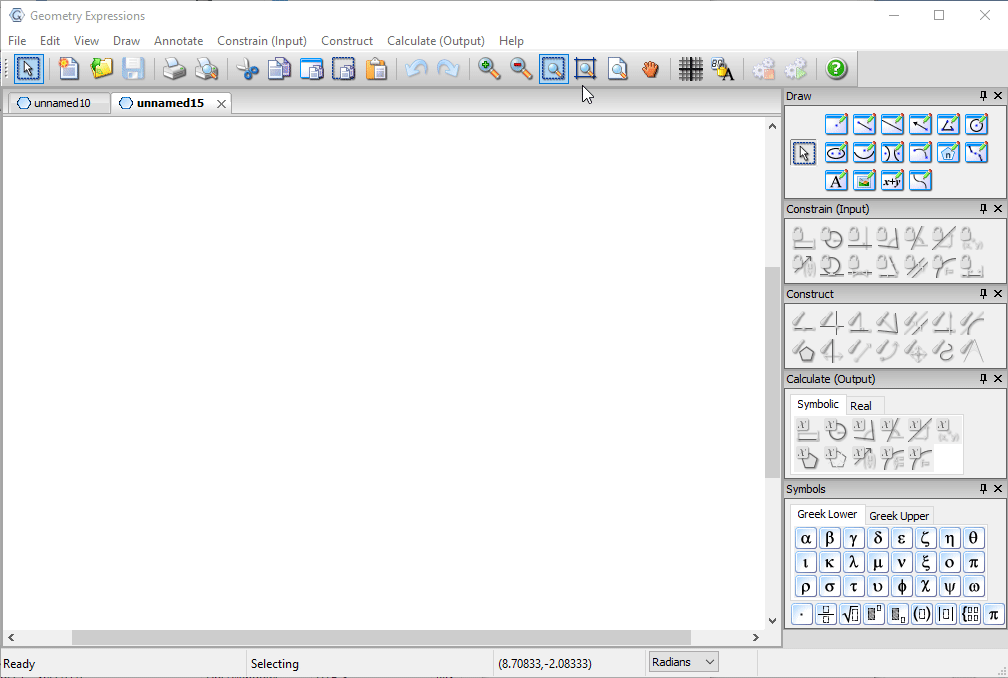Now create a tangent to the curve at parametric location t.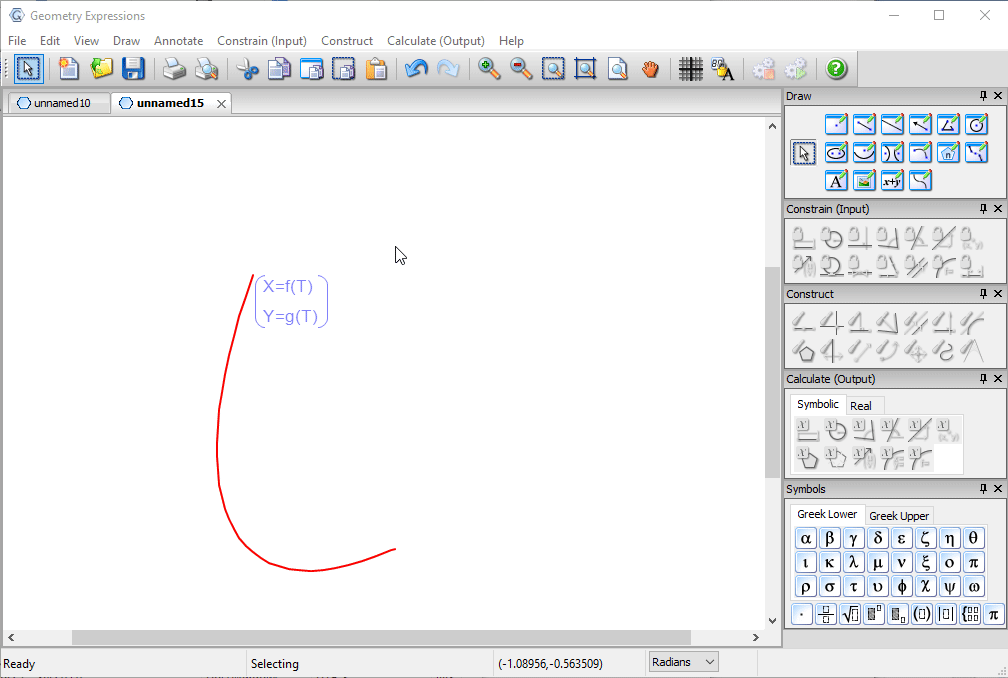Create the normal.

Construct the envelope of the normal as t varies. This is the evolute curve, the locus of the centers of curvature.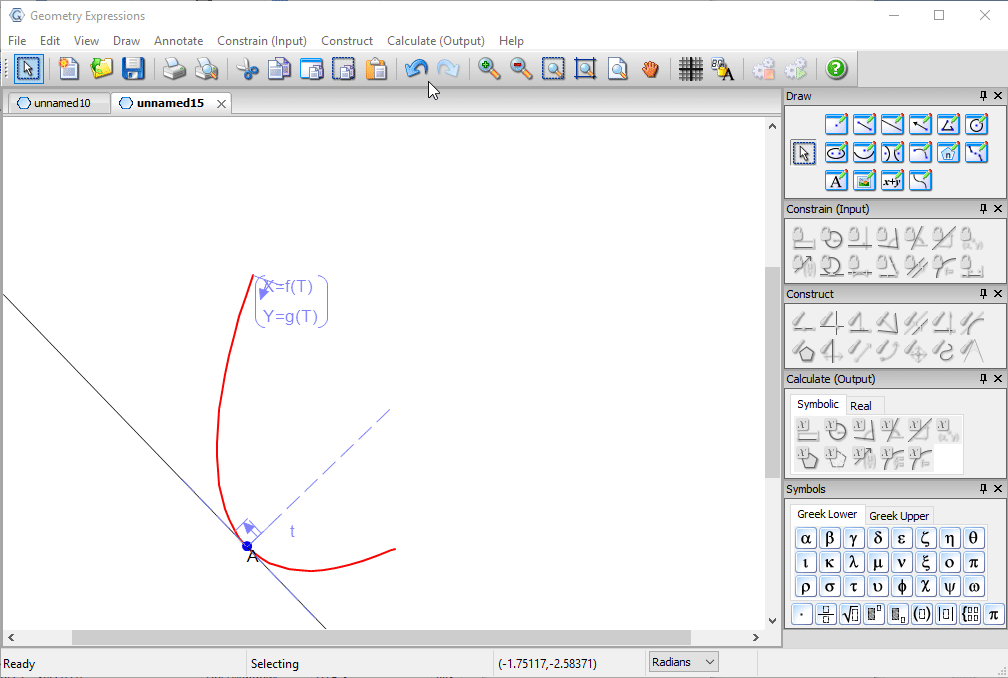Put a point on the evolute at parametric location t.Create the circle of curvature.View the coordinates of the center of curvature.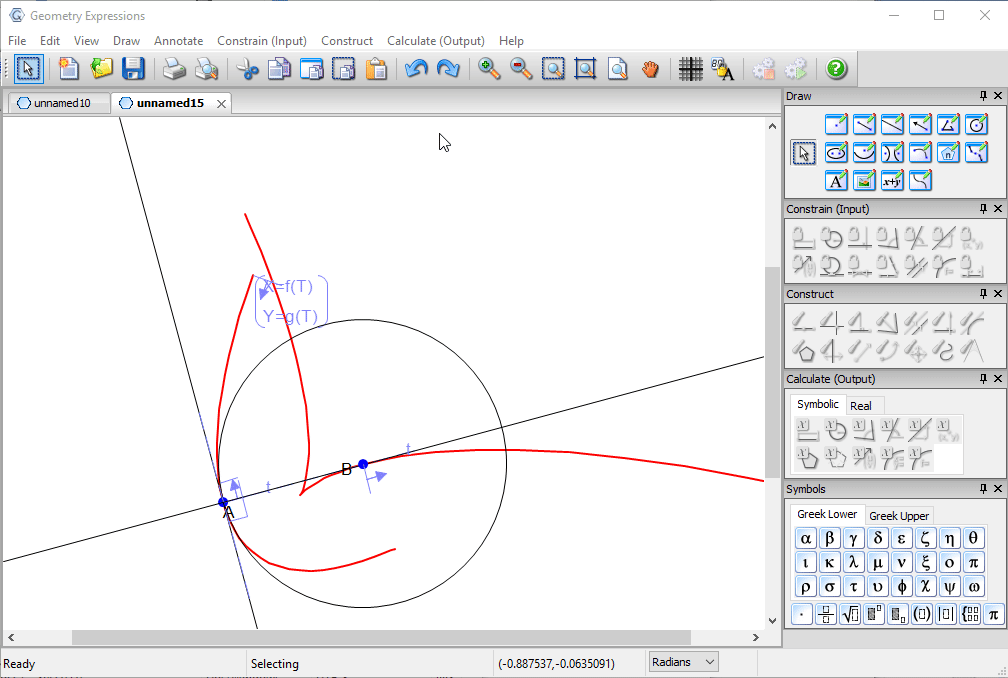Copy as Java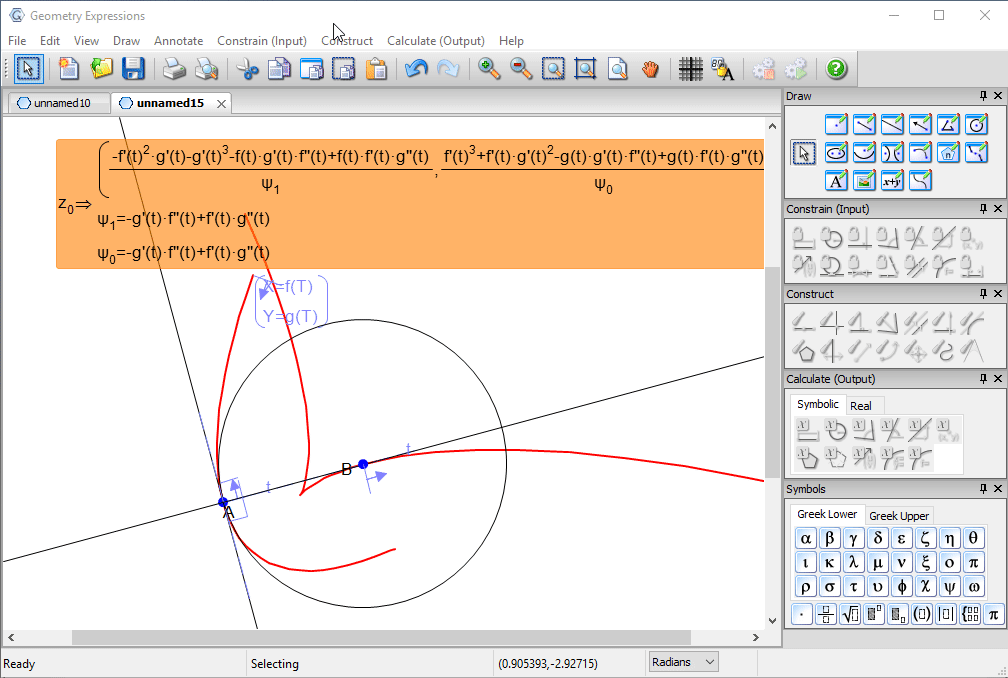And paste into a code editor.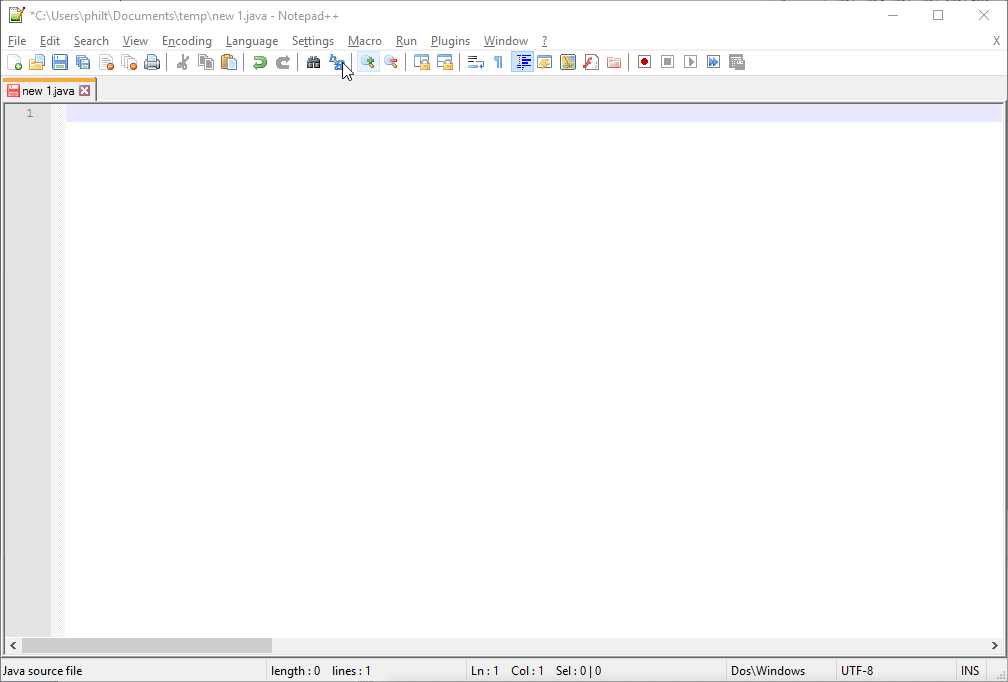Note we have placeholder generic functions f() and g() and numerical approximations to their first and second deivatives, which appear in the formula for the center of curvature.